## RS Aggarwal Class 10 Solutions Chapter 2 Polynomials Ex 2C

These Solutions are part of RS Aggarwal Solutions Class 10. Here we have given RS Aggarwal Class 10 Solutions Chapter 2 Polynomials Ex 2C. You must go through NCERT Solutions for Class 10 Maths to get better score in CBSE Board exams along with RS Aggarwal Class 10 Solutions.

Question 1.
Solution:
Let other zero of x2 – 4x + 1 be a, then
Sum of zeros = $$\frac { -b }{ a }$$ = $$\frac { -(-4) }{ 1 }$$ = 4
But one zero is 2 + √3
Second zero = 4 – (2 + √3) =4 – 2 – √3 = 2 – √3

Question 2.
Solution:
Let f(x) = x2 + x – p(p + 1)
= x2 + (p + 1) x – px – p(p + 1)
= x(x + p + 1) – p(x + p + 1)
= (x + p + 1) (x – p)
Either x + p + 1 = 0, then x = -(p + 1)
or x – p = 0, then x = p
Hence, zeros are p and -(p + 1)

Question 3.
Solution:
p(x) = x2 – 3x – m(m + 3)
= x2 – (m + 3)x + mx – m(m + 3)
= x(x – m – 3) + m(x – m – 3)
= (x – m – 3)(x + m)
Either x – m – 3 = 0, then x = m + 3
or x + m = 0, then x = -m
Zeros are (m + 3), -m

Question 4.
Solution:
a and p are the zeros of a polynomial
and α + β = 6, αβ = 4
Polynomial = x2 – (α + β)x + αβ = x2 – (6)x + 4 = x2 – 6x + 4

Question 5.
Solution:
One zero of kx2 + 3x + k is 2
x = 2 will satisfy it
⇒ k(2)2 + 3 x 2 + k = 0
⇒ 4k + 6 + k= 0
⇒5k + 6 = 0
⇒ 5k = -6
⇒ k = $$\frac { -6 }{ 5 }$$
Hence, k = $$\frac { -6 }{ 5 }$$

Question 6.
Solution:
3 is a zero of the polynomial 2x2 + x + k
Then 3 will satisfy it
2x2 + x + k = 0
⇒ 2(3)2 + 3 + k = 0
⇒ 18 + 3+ k = 0
⇒ 21 + k = 0
⇒ k = -21
Hence, k = -21

Question 7.
Solution:
-4 is a zero of polynomial x2 – x – (2k + 2)
Then it will satisfy the equation
x2 – x – (2k + 2) = 0
⇒ (-4)2 – (-4) – 2k – 2 = 0
⇒ 16 + 4 – 2k – 2 = 0
⇒ -2k + 18 = 0
⇒ 2k = 18
k = 9

Question 8.
Solution:
1 is a zero of the polynomial ax2 – 3(a – 1)x – 1
Then 1 will satisfy the equation ax2 – 3(a – 1) x – 1 = 0
a(1)2 – 3(a – 1) x 1 – 1 = 0
⇒ a x 1 – 3a + 3 – 1 = 0
⇒ a – 3a + 2 = 0
⇒ -2a + 2 = 0
⇒ 2a = 2
⇒ a = 1

Question 9.
Solution:
-2 is a zero of 3x2 + 4x + 2k
It will satisfy the equation 3x2 + 4x + 2k = 5
3(-2)2 + 4(-2) + 2k = 0
⇒ 3 x 4 + 4(-2) + 2k = 0
⇒ 12 – 8 + 2k = 0
⇒ 4 + 2k=0
⇒ 2k = -4
⇒ k = -2
k = -2

Question 10.
Solution:
Let f(x) = x2 – x – 6
= x2 – 3x + 2x – 6
= x(x – 3) + 2(x – 3)
= (x – 3)(x + 2)
(x – 3)(x + 2) = 0
Either x – 3 = 0, then x = 3
or x + 2 = 0, then x = -2
Zeros are 3, -2

Question 11.
Solution:
Sum of zeros = 1
and polynomial is kx2 – 3x + 5
Sum of zeros = $$\frac { -b }{ a }$$ = $$\frac { -(-3) }{ k }$$ = $$\frac { 3 }{ k }$$
$$\frac { 3 }{ k }$$ = 1
⇒ k = 3
Hence, k = 3

Question 12.
Solution:
Product of zeros of polynomial x2 – 4x + k is 3
Product of zeros = $$\frac { c }{ a }$$
⇒ $$\frac { k }{ 1 }$$ = 3
⇒ k = 3

Question 13.
Solution:
x + a is a factor of
f(x) = 2x2 + (2a + 5) x + 10
Let x + a = 0, then
Zero of f(x) = -a
Now f(-a) = 2 (-a)2 + (2a + 5)(-a) + 10 = 0
2a2 – 2a2 – 5a + 10 = 0
⇒ 5a = 10
⇒ a = 2

Question 14.
Solution:
(a – b), a, (a + b) are the zeros of 2x3 – 6x2 + 5x – 7
Sum of zeros = $$\frac { -b }{ a }$$
⇒ a – b + a + a + b = $$\frac { -(-6) }{ 2 }$$
⇒ 3a = $$\frac { 6 }{ 2 }$$
⇒ 3a = 3
⇒ a = 1

Question 15.
Solution:
f(x) = x3 + x2 – ax + 6 is divisible by x2 – x
Remainder will be zero
Now dividing f(x) by x2 – x
Remainder = (2 – a) x + b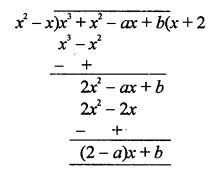(2 – a) x + b = 0
2 – a = 0
⇒ a = 2 and b = 0
Hence, a = 2, b = 0

Question 16.
Solution:
α and β are the zeros of polynomial f(x) = 2x2 + 7x + 5Question 17.
Solution:
Division algorithm for polynomials:
If f(x) and g(x) are any two polynomials with g(x) ≠ 0, then we can find polynomial q(x) and r(x).
f(x) = q(x) x g(x) + r(x)
where r (x) = 0
or [degree of r(x) < degree of g(x)]
or Dividend=Quotient x Division + Remainder

Question 18.
Solution:
Sum of zeros = $$\frac { -1 }{ 2 }$$
Product of zeros = -3
Polynomial: x2 – (Sum of zeros) x + product of zerosQuestion 19.
Solution: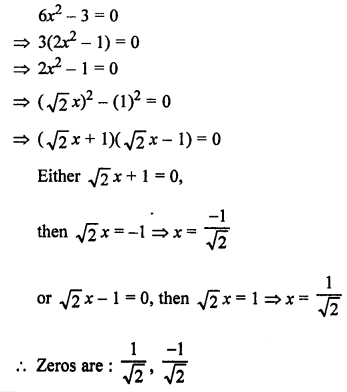Question 20.
Solution: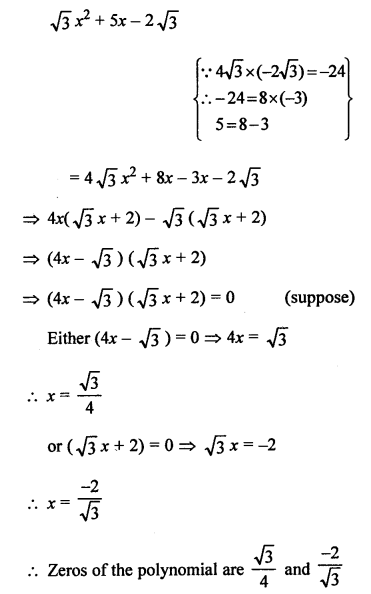Question 21.
Solution:
α and β are the zeros of polynomial f(x) = x2 – 5x + k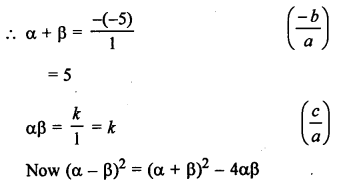(1)2 = (5)2 – 4 k
1 ⇒ 25 – 4k
⇒ 4k = 25 – 1 = 24
Hence, k = 6

Question 22.
Solution:Question 23.
Solution:
α and β are the zeros of polynomial
f(x) = 5x2 – 7x + 1Question 24.
Solution: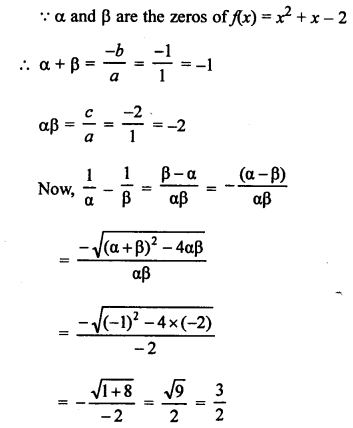Question 25.
Solution:
(a – b), a and (a + b) are the zeros of the polynomial
f(x) = x3 – 3x2 + x + 1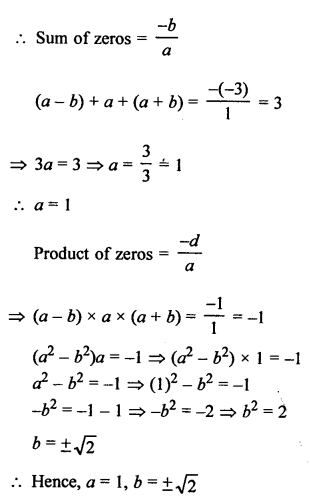Hope given RS Aggarwal Solutions Class 10 Chapter 2 Polynomials Ex 2C are helpful to complete your math homework.

If you have any doubts, please comment below. Learn Insta try to provide online math tutoring for you.